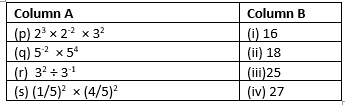# Exponents and powers class 8 worksheets with answers

In this page we have exponents and powers class 8 worksheets with answers .This worksheet has all format of questions covering the whole chapter. Questions format is Multiple choice questions, Match the column, Fill in the blank, short answer type. Hope you like them and do not forget to like , social share and comment at the end of the page.

Question 1
Evaluate
1. 2-2
2. (-2)-2
3. (3/2)-5

Question 2
Simplify and express the result in power notation with positive exponent.
(i) (-2)5 ÷ (-2)4
(ii) (1/2)2 × (2/5)2
(iii) (-5)2 × (3/5)

Question 3
Find the value of.
(i)(40 + 4-1) × 22
(ii)(3-1 × 9-1) ÷ 3-2
(iii)(11-1 + 12-1 + 13-1)0

Question 4
Find the value of x here
$( \frac {11}{9})^3 \times (\frac {9}{11})^6 = (\frac {11}{9})^{2x-1}$

Question 5
Find the value of m for which 2m ÷ 2-4 = 45

Question 6
Express the following numbers in standard form.
(i) 0.0000000015
(ii) 0.00000001425
(iii) 102000000000000000

Question 7
Express the following numbers in usual form.
(i) 34.02 x 10-5
(ii) 9.5 x 105
(iii) 9 x 10-4
(iv) 2.0001 x 108

Question 8
Solve for the variables
(i) $\frac {1}{y^{-5}} = 32$
(ii) $(\frac {1}{7})^3 \div (\frac {1}{7})^8 = 7^n$
(iii) $\frac {3^x .3}{3^{2x+1}} = 27$

Question 9
Find the Multiplicative inverse of
(i) $3^{25}$
(ii) $4^{-3}$
(iii) $(\frac {2}{3})^{-2}$

Question 10
Simplify the following
(i) $1 + \left [ \left ( \frac{1}{3} \right )^{-3} - \left ( \frac{1}{2} \right )^{-3} \right ] \div 38$
(ii) $\frac {1}{1 + p^{a-b}} + \frac {1}{1 + p^{b-a}}$

## True and false

Question 11
True and False
(i) Very small numbers can be expressed in standard form using negative exponents.
(ii) $a^p \times b^q= (ab)^{pq}$
(iii) $.00567 = 5.67 \times 10^{-3}$
(iv) $\frac {1}{(8)^{-3}}= 2^9$
(v) $4^0 =4$
(vi)$(-1)^3=1$
(vii) The multiplicative inverse of $(–2)^{–2}$ is $(2)^2$.

## Fill in the blanks

Question 12
Fill in the blank
(i) The multiplicative inverse of $(3)^{–2}$ is _____
(ii) $9^7 \times 3^{-2}$=_____
(iii) .0000067 in exponent form _____
(iv) Very large numbers can be expressed in standard form by using_________ exponents
(v) $5^0$ =____
(vi)$(-1)^100$=____
(vii) The value of $[4^{–1} +3^{–1} + 6^{–2}]^{–1}$ is ____.

## Match the column

Question 13## Multiple choice questions

Question 14
If a be any non-zero integer, then $a^{–1}$ is equal to
(a) a
(b) -a
(c) 1/a
(d) -1/a
Question 15
The multiplicative inverse of $(–7)^{–2} \div (90)^{–1}$ is
(a) $-(7)^2 \times (90)^{–1}$
(b) $-(7)^{-}2 \times (90)^{–1}$
(c) $-(7)^2 \div (90)^{–1}$
(d) $-(7)^2 \times (90)^{1}$
Question 16
If $5^{3x–1} \div 25 = 125$, Then the value x is
(a) 1
(b) 2
(c) 3
(d) 4

## Summary

This exponents and powers class 8 worksheets with answers is prepared keeping in mind the latest syllabus of CBSE . This has been designed in a way to improve the academic performance of the students. If you find mistakes , please do provide the feedback on the mail.